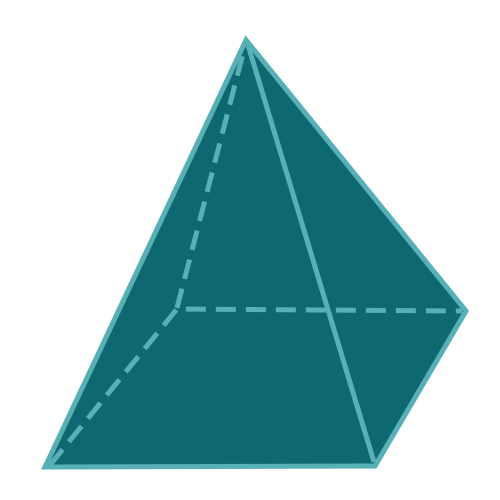Report a Problem
Suggestions

#Calculate The Volume of A Pyramid With A Square Base

Last updated: Saturday, June 24, 2023
Select a type of pyramid below
Rectangular Base
Square Base
Equilateral Triangular Base
Tetrahedron
Pentagonal Base
Hexagonal Base

A pyramid with a square base is a polyhedron consisting of a flat, square base and four triangular faces that converge at a single apex. This classic pyramid shape is both aesthetically pleasing and structurally sound, making it a popular choice in various fields.

In the real world, the pyramid with a square base is perhaps best exemplified by the iconic Great Pyramids of Egypt. This shape is also used in modern architecture, such as the Louvre Pyramid in Paris. In addition, smaller decorative items like paperweights or ornaments may adopt this form for its visual appeal and stability.

Easily calculate the volume of a pyramid with a square base with step-by-step guidance using our free calculator below.

The formula for determining the volume of a pyramid with a square base is defined as:
$$V$$ $$=$$ $$a^2 \cdot \dfrac{h}{3}$$
$$V$$: the volume of the pyramid
$$a$$: the length of of any side of the base
$$h$$: the height of the pyramid
The SI unit of volume is: $$cubic \text{ } meter\text{ }(m^3)$$

## Find $$V$$

Use this calculator to determine the volume of a pyramid with square base using the base area and the height.
Hold & Drag
CLOSE
the length of of any side of the base
$$a$$
$$meter$$
the height of the pyramid
$$h$$
$$meter$$
Bookmark this page or risk going on a digital treasure hunt again